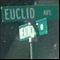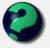# Math Is Fun Forum

Discussion about math, puzzles, games and fun.   Useful symbols: ÷ × ½ √ ∞ ≠ ≤ ≥ ≈ ⇒ ± ∈ Δ θ ∴ ∑ ∫  π  -¹ ² ³ °

You are not logged in.

## #1 2008-10-16 16:10:56

fusilli_jerry89
Member
Registered: 2006-06-23
Posts: 86

### Weird Proof

Consider the following theorem:
A positive integer n is divisible by 2^t
if and only if the number consisting of the
last t digits of n is divisible by 2^t

For instance 68 is divisible by 2^2 = 4, and hence 38168 is divisible by 4. On the other hand,
0260 is not divisible by 2^4 = 16, and so 10440260 is not divisible by 16.

Prove the theorem using a direct proof. Hint: to prove a biconditional, you need to
prove two implications.

Yeah Im confused..

Offline

## #2 2008-10-16 16:40:58

Ricky
ModeratorRegistered: 2005-12-04
Posts: 3,791

### Re: Weird Proof

How many powers of 2 are contained in 10^t?  How many powers of 10 are contained in t-digits?

"In the real world, this would be a problem.  But in mathematics, we can just define a place where this problem doesn't exist.  So we'll go ahead and do that now..."

Offline

## #3 2008-10-16 16:53:55

fusilli_jerry89
Member
Registered: 2006-06-23
Posts: 86

### Re: Weird Proof

so im a bit rusty:

yer asking (10^t)/2^x ; what is x right? Ok well I forgot how to divide powers with different bases lol.

Offline

## #4 2008-10-16 17:17:13

fusilli_jerry89
Member
Registered: 2006-06-23
Posts: 86

### Re: Weird Proof

ok well apparently im very confused. You cant divide power with different powers, right?
So how am i supposed to find how many powers of 2 are in 10^t ?

Offline

## #5 2008-10-16 19:05:10

fusilli_jerry89
Member
Registered: 2006-06-23
Posts: 86

### Re: Weird Proof

lol i hate to be annoying but im kinda in a hole here. I have a midterm tomorrow on this stuff and I found that I get almost everything except this kind of question. The prof said a similar question would be on the exam but i was sick and missed the solution. Is there any way I can get quick help before tomorrow before 12:30? Thx

Offline

## #6 2008-10-16 20:42:31

fusilli_jerry89
Member
Registered: 2006-06-23
Posts: 86

### Re: Weird Proof

ok so I said that n=the number, and the number t is the amount of numbers at the end of n. I call the numbers in t x. so n = x + (n - x) (where x = last digits). So now I must show that 2^t | n implies 2^t | the last digits and 2^t | the last digits implies 2^2 | n.

So n = x + y10^t (where y is the first digits and x is the last digits)

So now I think I must show that 2^t divides n and 2^t divides (x + y10^t).

Now I am stuck..

Offline

## #7 2008-10-17 03:05:07

mathsyperson
ModeratorRegistered: 2005-06-22
Posts: 4,900

### Re: Weird Proof

You're getting there, but I think you've confused yourself about what you need to prove.
You've defined n = x + y*10^t, with x being the last t digits of n.

Then, the original question in terms of these is to prove that:
2^t divides x if and only if 2^t divides (x + y*10^t).

You do NOT need to prove that those two things are true (that would be wrong), just that they're always both true or both false.

The question hints that you can do this by showing each implication separately.
ie. Assume that 2^t divides x and use that to show that 2^t divides (x + y*10^t).
Then do it the other way around. I'm not sure you need to do that, since both of those proofs would be pretty similar, but it's something to think about if you're still stuck.

Why did the vector cross the road?
It wanted to be normal.

Offline

## #8 2008-10-17 06:13:14

fusilli_jerry89
Member
Registered: 2006-06-23
Posts: 86

### Re: Weird Proof

k but if we assume 2^t divides n and try to prove that 2^t divides x + y10^t , can't we just say that since n = x + y10^t and 2&t divides n than it must divide x + y10^t because that's what n is?

Or must I do more than this? lol

Offline

## #9 2008-10-17 07:20:51

fusilli_jerry89
Member
Registered: 2006-06-23
Posts: 86

### Re: Weird Proof

10 mins till exam..

Offline

## #10 2008-10-17 07:37:19

bitus
Member
Registered: 2008-10-12
Posts: 20

### Re: Weird Proof

Let n be:a(t+k)a(t+k-1)a(t+k-2)...a(t+1)a(t)a(t-1)...a(2)a(1). It has t+k digits. The last t digits form a t-digit number divided by 2^t. The first k digits form a k-digit number that can be written as : 10^[t+1]*[a(t+k)*10^[k]+a(t+k-1)*10^[k-1]+...+a(t+1)]. This number is divided by 2^t since 10^[t+1] is divited by 2^t: 10^[t+1]=[2*5]^[t+1]=2^t*[2*5^[t+1]]. So, the whole n is divited by 2^t. If the t-digit number is not divided by 2^t, then (n/2^t) is not an integer.

Offline